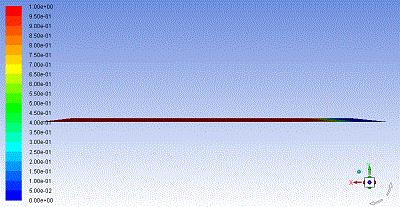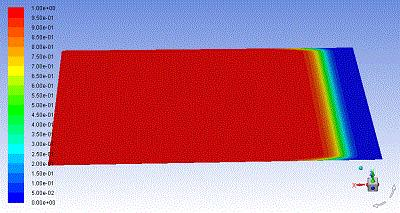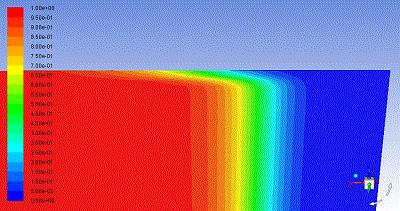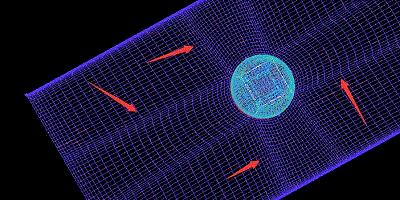## Fluids

•h395523899
Subscriber

1. My model is 20m*10m*10mm. In the initial state, the model is all oil.

Water enters from one end at a speed of 5m/s, and the other is a free exit.

Euler model, k-e turbulence model was used. The default state for all others.

2. The water breaks through the oil at the model boundary in the simulation. The figure shows the volume fraction cloud of the oil phase. The blue area is water.

However, according to the situation in the literature, it should be the first breakthrough of water in the middle part of the model. The whole water phase (blue part) should be tapered, but the simulated image is the opposite•h395523899
Subscriber

This was not the case when I simulated the same setup with a 5m*5m*10mm model.

The volume fraction of water in the model boundary is slightly less than the middle part. There was no situation where the water at the border first took away the oil phase.

•raul.raghav
Subscriber
The contours don’t look right as you mention. Would you be able to share your workbench archive file?
•h395523899
Subscriber

1. I didn't use the workbench. I drew the mesh with icem and then opened it with fluent. Because I'm not very proficient with the workbench.

2. I seem to find the reason. Because my grid size gap is too big, or that the boundary is not dense.

Problem Model: I set 11 nodes on the edge of 10mm, and set 201 nodes for each dimension of 1.20m, and each spacing is 100. The 10m edge sets 101 nodes, each spacing size 100.

Modified model: The nodes and dimensions on the 10mm edge are unchanged. The 20m side sets both sides of the spacing dimension 1, and the rate of change is 1.25. Similarly, the 10m edge is also modified. With the node unchanged, the grid max space of the 10m and 20m edges was adjusted.

If it is the size of the grid, then I have a few questions I would like to ask you.

1. For this model containing 20m-10mm dimensions, how many nodes can be set to ensure the least impact on accuracy? For example, the mesh size of the 10mm edge is taken as 1, 0.1, 0.01... The smaller the better?

2. how much is the size changing rate (Ratio) set for grid encryption? According to some videos, I know that some are set to 1.25, others are set to 1.2. What is the basis for this setting?

3. Can I know how much memory is needed to run in fluent based on the number of structured grids? Because my RAM is not very large, there are limits to the number of grids. It is also possible that the graphics card will be overloaded after a large number of grids.

•h395523899
Subscriber

•raul.raghav
Subscriber

The 10mm thickness would cause you issues. Have you considered 2D planar setup for this problem? Then the mesh size would be a lot more easier to manage since you mention that you are restricted by the RAM. You can define 201 nodes on both the 20m and 10m sides to begin with. The 10m side would require a finer mesh near the walls. The flow direction can have less number of nodes because the velocity gradient in the flow direction would be small.

1. This largely depends on the flow velocity as well on the physics of the flow (multiphase, buoyancy etc). This is where mesh convergence and independence studies come into the picture. You start off with a certain mesh size and successively increase it till you get a mesh size where your flow variables do not change. Smaller is better but not always. The smallest mesh size which can provide the best possible results is what we aim for.

2. In general for CFD, a growth rate of 1.1 to 1.3 is recommended. I would stick to the defaults for simple geometries. Can you attach a schematic of your mesh? I'm assuming that its hexa mesh that you're working with.

3. I believe 1 to 1.2 million should work fine with 4gb RAM.

Uploading your files: If you have your geometry, mesh and fluent files separately, I'd say zip the files and attach them. If the size is larger than 120mb, use google drive to share the files.

•h395523899
Subscriber

Lf-rd is an icem file +fluent file.

There is also an lf is icem file. The fluent setting used in lf is the same as in lf-rd.

What I ultimately want to simulate is the model in lf. One plate plus two hemispheres.

Model size: length 20m, width 10m, thickness 10mm, two hemispherical diameter 13m. Water enters from the surface of 10m*10mm and flows out from the other end. In the initial state, the model is oil.

In the past few days I have encountered several problems. Please give pointers.

1. Regarding wall mesh encryption, the wall distance, y+, and wall function are mentioned. What is their relationship, I didn't figure it out. In particular, seeing that the first grid is at the y+ =1 position, the grid I calculated would be very dense and could not be implemented at all.

2. I see a way to use y+ between 30 and 300 when using the standard wall function.

What if you see y+ is less than 30 or greater than 300 in post-processing?

3. In my lf file, do I not need mesh encryption at the junction of sheet and hemisphere? If needed, how does this wall distance be calculated?

4. Encrypting the part of the sheet that I contact with the ball will make it encrypted elsewhere, which seems to affect my simulation. Please look at the picture.............•h395523899
Subscriber

Lf-rd is an icem file +fluent file.

There is also an lf is icem file. The fluent setting used in lf is the same as in lf-rd.

What I ultimately want to simulate is the model in lf. One plate plus two hemispheres.

Model size: length 20m, width 10m, thickness 10mm, two hemispherical diameter 13m. Water enters from the surface of 10m*10mm and flows out from the other end. In the initial state, the model is oil.

In the past few days I have encountered several problems. Please give pointers.

1. Regarding wall mesh encryption, the wall distance, y+, and wall function are mentioned. What is their relationship, I didn't figure it out. In particular, seeing that the first grid is at the y+ =1 position, the grid I calculated would be very dense and could not be implemented at all.

2. I see a way to use y+ between 30 and 300 when using the standard wall function.

What if you see y+ is less than 30 or greater than 300 in post-processing?

3. In my lf file, do I not need mesh encryption at the junction of sheet and hemisphere? If needed, how does this wall distance be calculated?

4. Encrypting the part of the sheet that I contact with the ball will make it encrypted elsewhere, which seems to affect my